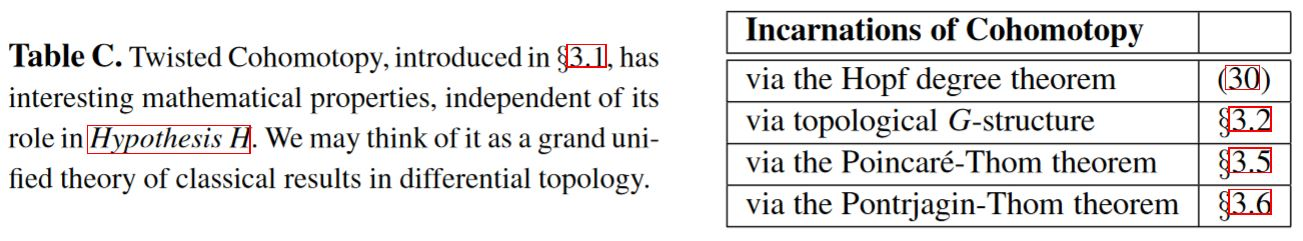# nLab twisted cohomotopy

Contents

cohomology

## Theorems

#### Spheres

n-sphere

low dimensional n-spheres

# Contents

## Idea

Twisted cohomotopy is the twisted cohomology-variant of the the non-abelian cohomology-theory cohomotopy, represented by homotopy types of n-spheres.

The coefficients/twist for twisted cohomotopy are spherical fibrations, and cocycles are sections of these. For those spherical fibrations arising as unit sphere bundles of real vector bundles the twist may be understood as given by the J-homomorphism.

## Properties

Various classical theorems of differential topology are secretly theorems about twisted cohomotopytable grabbed from FSS 19b

### Twisted Pontrjagin theorem

The twisted Pontrjagin theorem relates twisted cohomotopy with cobordism classes of normally twisted-framed submanifolds.

### Twisted May-Segal theorem

The May-Segal theorem has a twisted generalization:

###### Proposition

Let

1. $X^n$ be a smooth closed manifold of dimension $n$;

2. $1 \leq k \in \mathbb{N}$ a positive natural number.

Then the scanning map constitutes a weak homotopy equivalence

$\underset{ \color{blue} { \phantom{a} \atop \text{ J-twisted cohomotopy space}} }{ Maps_{{}_{/B O(n)}} \Big( X^n \;,\; S^{ \mathbf{n}_{def} + \mathbf{k}_{\mathrm{triv}} } \!\sslash\! O(n) \Big) } \underoverset {\simeq} { \color{blue} \text{scanning map} } {\longleftarrow} \underset{ \mathclap{ \color{blue} { \phantom{a} \atop { \text{configuration space} \atop \text{of points} } } } }{ Conf \big( X^n, S^k \big) }$

between

1. the J-twisted (n+k)-cohomotopy space of $X^n$, hence the space of sections of the $(n + k)$-spherical fibration over $X$ which is associated via the tangent bundle by the O(n)-action on $S^{n+k} = S(\mathbb{R}^{n} \times \mathbb{R}^{k+1})$

2. the configuration space of points on $X^n$ with labels in $S^k$.

(Bödigheimer 87, Prop. 2, following McDuff 75)

###### Remark

In the special case that the closed manifold $X^n$ in Prop. is parallelizable, hence that its tangent bundle is trivializable, the statement of Prop. reduces to this:

Let

1. $X^n$ be a parallelizable closed manifold of dimension $n$;

2. $1 \leq k \in \mathbb{N}$ a positive natural number.

Then the scanning map constitutes a weak homotopy equivalence

$\underset{ \color{blue} { \phantom{a} \atop \text{ cohomotopy space}} }{ Maps \Big( X^n \;,\; S^{ n + k } \Big) } \underoverset {\simeq} { \color{blue} \text{scanning map} } {\longleftarrow} \underset{ \mathclap{ \color{blue} { \phantom{a} \atop { \text{configuration space} \atop \text{of points} } } } }{ Conf \big( X^n, S^k \big) }$

between

1. $(n+k)$-cohomotopy space of $X^n$, hence the space of maps from $X$ to the (n+k)-sphere

2. the configuration space of points on $X^n$ with labels in $S^k$.

See at

### Equivariant Hopf degree theorem

On flat orbifolds, twisted cohomotopy becomes equivariant cohomotopy and the twisted Hopf degree theorem becomes the

flavours of
Cohomotopy
cohomology theory
cohomology
(full or rational)
equivariant cohomology
(full or rational)
non-abelian cohomologyCohomotopy
(full or rational)
equivariant Cohomotopy
twisted cohomology
(full or rational)
twisted Cohomotopytwisted equivariant Cohomotopy
stable cohomology
(full or rational)
stable Cohomotopyequivariant stable Cohomotopy
differential cohomologydifferential Cohomotopyequivariant differential cohomotopy
persistent cohomologypersistent Cohomotopypersistent equivariant Cohomotopy

The concept is implicit in classical texts on differential topology, for instance

Discussion of the twisted Pontrjagin theory? and of twisted stable cohomotopy (framed cobordism cohomology theory):

Discussion of unstabilized twisted cohomotopy, with application to foundations of M-theory (Hypothesis H):